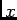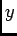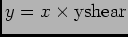# OOF: Finite Element Analysis of Microstructures

/initialize/uniform variablesNext: /modify/replace Up: /initialize/uniform Previous: /initialize/uniform/isotropic, etc.   Contents

## /initialize/uniform variables

nx
The number of element edges to create in the(horizontal) direction. Default: 20.

ny
The number of element edges to create in the(vertical) direction. Default: 20.

width
The width of the rectangle in the same system of units that elastic parameters will be specified. The thickness (out-of-screen) physical dimension is assumed to be one, so all results (or scale moduli appropriately) should be interpreted with this in mind. Default: 1.

See section 4.4 for more discussion about units.

height
The height of the rectangle in physical units. Default: 1.

diagonals
A choice variable for how to draw the diagonals in each rectangle. Choices are: liberal (all diagonals point to the left); conservative (all diagonals point to the right); moderate (half liberal, half conservative); and anarchic (randomly assigned diagonals); Default: liberal

interior_nodes
A choice variable for which types of degrees of freedom are allowed at the interior nodes. Choices: 1) xy (both degrees of freedom) 2) linear (allow movement along a general line only. We once thought this was useful but have forgotten why. The linear node maintains its own coordinate system. Initially the nodes'andaxes are lined up with the screen axes, but when a shear distortion is applied the axes rotate (Section 3.27). The boundary conditions are applied in the node's coordinate system (Section 3.6). For example, if the nodes on the bottom edge of the grid are linear nodes, a yshear distortion has been applied, and the nodes have been fixed in y, then during equilibration they can move only along the line. ) Default: xy

boundary_nodes
A choice variable for which types of degrees of freedom are allowed at the boundary (edges of the rectangle) nodes. Same choices as interior_nodes. Default: xyNext: /modify/replace Up: /initialize/uniform Previous: /initialize/uniform/isotropic, etc.   Contents
/* Send mail to the OOF Team *//* Go to the OOF Home Page */